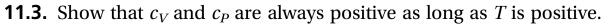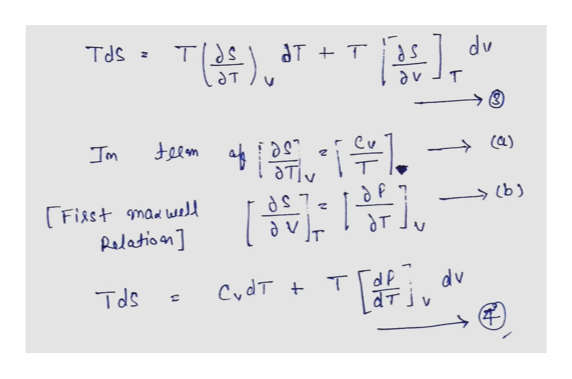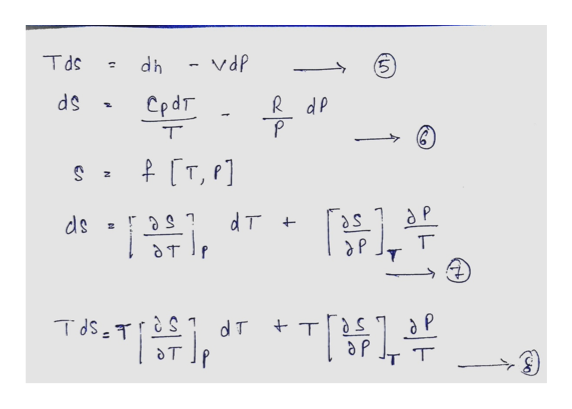# 11.3. Show that cy and cp arealways positiveas T is positive.longas

Question

This problem is (11.3) from a book  "Thermodynamics and Statistical Mechanics An Integrated Approach by M. Scott Shell"help_outlineImage Transcriptionclose11.3. Show that cy and cp are always positive as T is positive. long as fullscreen
check_circleExpert Solution
Step 1

By helping  to the first law of thermodynamic equation 1 is converted in terms of CV and ideal gas law.

After converting equation 1 result equation 2 because enthalpy is the function of f (T, V).

Step 2

Equation 2 multiply by T and get equation 3, in terms of enthalpy at constant volume converted into CV/T sub-equation (a) and first Maxwell relation sub-equation (b). Put in the equation 3.

Found that first TdS relation equation 4.help_outlineImage TranscriptioncloseTds= TS dT T T Ca) Fisst mawl Pslatioon LSP vT dP CydT T dv Tds fullscreen
Step 3

Behalf of third Maxwell relation got that equation 5, arranging the equation in terms of temperature equation 6 write that. ...help_outlineImage TranscriptioncloseTds dPA-4p R dP P dP CpdT T [T, F] z ds dT = S T T dsT T S d T T T T CA fullscreen

### Want to see the full answer?

See Solution

#### Want to see this answer and more?

Solutions are written by subject experts who are available 24/7. Questions are typically answered within 1 hour*

See Solution
*Response times may vary by subject and question
Tagged in

### Chemical Engineering